# GMAT Math : Counting Methods

## Example Questions

← Previous 1 3 4

### Example Question #1 : Understanding Counting Methods

In how many ways can the 11th grade class elect a president, vice president, and treasurer from a class of 70 students?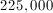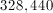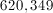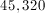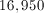Explanation:

The president can be elected in 70 different ways. After a student is elected president, there are 69 students left to elect a vice president from. Similarly, there are then 68 students left for the spot of treasurer. So there are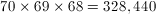different arrangements.

### Example Question #1 : Understanding Counting Methods

What is the number of possible 4 letter code words that can be made from the alphabet, when all 4 letters must be different?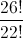4!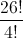26!

22!Explanation:

This is a permutation of 26 objects (letters) taken 4 at a time.  Here order matters, because for example, "abcd" is not the same code word as "bdca".

You must know the permutation formula!  It is as follows: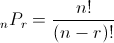, where n is the number of different objects taken r at a time.

Here we have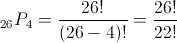Note: This is equivalent to 26 * 25 * 24 * 23.

### Example Question #1 : Understanding Counting Methods

There are 8 paths between placesand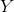and 5 paths betweeen placesand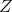. How many different routes are there between placesand?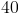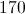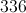Explanation:

Multiple the number of routes for each piece of the trip: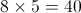### Example Question #1 : Understanding Counting Methods

How many subsets does a set with 12 elements have?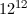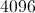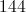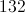Explanation:

The number of subsets in a set of sizeis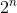. If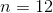, then the set has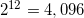subsets.

Alternatively, each subset of this twelve-element set is essentially a sequence of 12 independent decisions, one per element - each decision has two possible outcomes, exclusion or inclusion. By the multiplication principle, this is 2 taken as a factor 12 times, or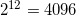### Example Question #1 : Understanding Counting Methods

How many ways can a president, a vice-president, a secretary-treasurer, and three Student Senate representatives be selected from a class of thirty people? You may assume these will be six different people.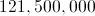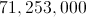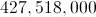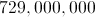Explanation:

This can be seen, without loss of generality, as choosing each officer in turn.

There are 30 ways of choosing the president; there are then 29 ways of choosing the vice-president, and 28 ways of choosing the secretary-treasurer. Then 3 Student Senate representatives are chosen from the remaining 27 students; this is a combination of 3 elements from 27 - that is,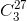. By the multiplication principle, the number of possible selections of the officers is: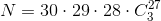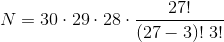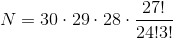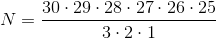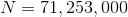### Example Question #6 : Understanding Counting Methods

How many ways can you select three different prime numbers between 1 and 20?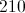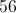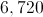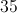Explanation:

There are eight prime numbers between 1 and 20: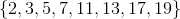The number of ways to select three of them, without regard to order, is the number of combinations of three out of eight: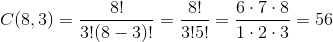### Example Question #1 : Understanding Counting Methods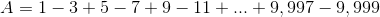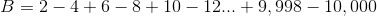Which of the following statements is true?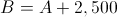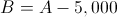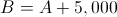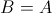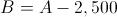Explanation:

The finite seriesis obtained fromby increasing each term by 1; sinceis an alternating series, this results in adding to: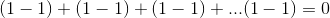so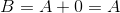### Example Question #1 : Counting Methods

Define set.

How many four-element subsets ofinclude at least three even numbers?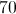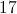Explanation:

Only one subset ofhas four even numbers - that is the subset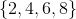.

Forming a subset with three even numbers and one odd number can be restated as choosing which even number to leave out and which odd number to include. There are 4 choices each way, so the number of sets fitting this description is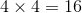.

Therefore, the number of subsets with at least three even elements is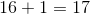.

### Example Question #9 : Understanding Counting Methods

Twelve students are running for student council; each student will vote for four. Mick wants to vote for his sister Janine. How many ways can he cast his ballot so as to include Janine among his choices?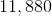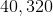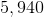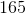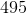Explanation:

Since one of Mick's choices is already decided, he will choose three people from a set of eleven without regard to order. This is a combination of three from a set of eleven; the number of such combinations is: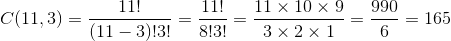### Example Question #1 : Counting Methods

Twelve students are running for student council; each student will vote for five. Claude does not want to vote for Gary or Mitch, neither of whom he likes. How many ways can Claude fill in the ballot so that he does not vote for Gary?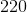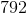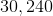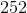Explanation:

Claude will choose five people from a set of ten - twelve minus the two he dislikes - without regard to order. This is a combination of five from a set of ten; the number of such combinations is: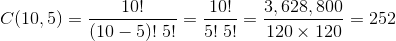← Previous 1 3 4

### All GMAT Math Resources